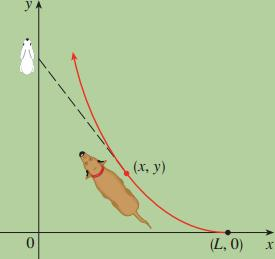# 100 followers special

Calculus Level 5A dog sees a rabbit running in a straight line across an open field and gives chase. In a rectangular coordinate system (as shown in the figure), assume:

(i) The rabbit is at the origin and the dog is at the point $(L,0)$ at the instant the dog first sees the rabbit.

(ii) The rabbit runs up the $y$-axis and the dog always runs straight for the rabbit.

(iii) The dog runs at twice the speed of the rabbit.

Assuming $L=300$ units, how much does the dog have to travel to catch the rabbit?

Enter your answer as the distance the dog has traveled from sight to meal.

×

Problem Loading...

Note Loading...

Set Loading...